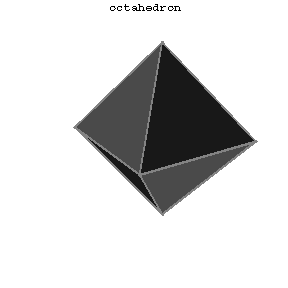# Octahedron

A solid figure having eight triangular faces, twelve edges and six vertices, with 4 faces at each vertex. If all edges have the same length, it is one of the five regular polyhedra (Platonic solids); if the edge length is $a$, then the volume of the octahedron is

$$v=\frac{a^3\sqrt 2}{3}\approx0.4714a^3.$$Figure: o068100a

The Schläfli symbol of an octahedron is $\{3,4\}$. When the edges all have the same length one deals with the regular octahedron, reciprocal to the cube; it can be regarded either as a triangular anti-prism or as a square double-pyramid. As one of the five Platonic polyhedra (cf. Regular polyhedra; Platonic solids) it represents the ancient element air. It occurs in nature as a crystal of chrome alum. In terms of its circumradius as unit of measurement, its six vertices have Cartesian coordinates
$$(\pm1,0,0),\quad(0,\pm1,0)\quad(0,0,\pm1);$$
thus it has edge-length $\sqrt2$, inradius $\sqrt{1/3}$ and volume $4/3$. Its 4 pairs of opposite faces (or the 4 diameters of the cube) are freely permuted by the octahedral group $\mathfrak S_4$ of order $4!=24$.